# Average and Range Method Gage R&R HelpThis help page shows how to setup and run the Average and Range method Gage R&R for a crossed design.  This is the design where multiple operators repeatably measure multiple parts. The data used in this example can be downloaded at this link.

1. To start the analysis, select “MSA” from the “Analysis” panel on the SPC for Excel ribbon.  Then select “Gage R&R Studies” and “Crossed-Average/Range Method”.

2. The input form below is shown.  Enter the number of operators, parts and trials.  You also have the option to randomize the runs in the study. Select OK.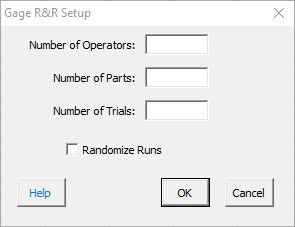3. The option to enter the operators’ names is given.  If you select No, the software lists each operator as Operator 1, Operator 2, etc.  If you select yes, then an input form is shown for each operator so you can enter the name.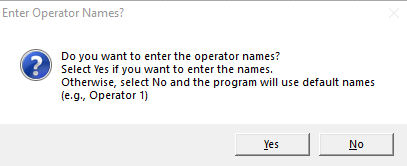4. An Average/Range Method Gage R&R worksheet is added to the workbook. This is where you enter the information and results.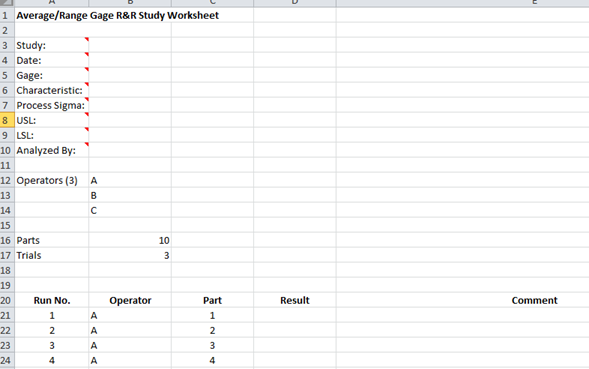5. Enter the information into the top part of the worksheet.  There is a comment in each cell in rows 3 to 10 in column A that explain the entry. All entries are optional.  Each entry is explained below.

• Study: optional,name of study
• Date: optional, date Gage R&R performed
• Gage: optional, name of gage
• Characteristic: optional, characteristic being measured
• Process Sigma: optional, value used for the total variance instead of the calculated total variance of the Gage R&R results
• USL: optional, used to determine % of tolerance explained by study variation
• LSL: optional, used to determine % of tolerance explained by study variation
• Analyzed By: optional, name of person doing the analysis

6. Enter the run number, the sample result for each combination of operator and part and any comments (optional).

7. Part of a completed Average/Range worksheet is shown below.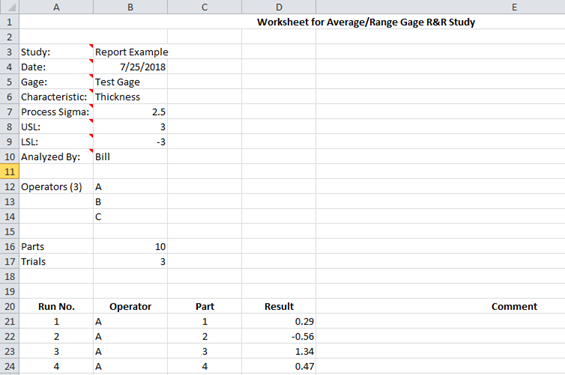8. The results are now ready to be analyzed using the average/range method. To analyze the results, select “MSA” from the “Analysis” panel on the SPC for Excel ribbon.

9. The following options are displayed.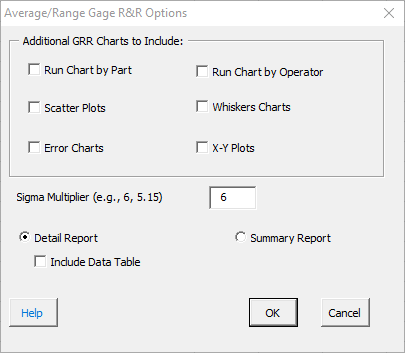There are six additional charts that can be created.  These are shown in the input form above. There is also the sigma multiplier – the value that standard deviation is multiplied by. The default value is 6.  This captures 99.73% of the data.  5.15 is sometimes used. This value captures 99% of the data.  You also have an option for detailed report or a summary report, both displayed on single worksheet. The detailed report contains all the charts, calculations and explanation of results.  The summary report contains the charts.  You also have the option to include a data table in the report.  Select OK to generate the report in the workbook.  Any charts selected are placed on a separate worksheet.

Select OK to generate the report in the workbook.

10. A pdf file containing the detailed report with a description of the output is given at this link.  The output includes:

• % Contributions based on standard deviation
• % Contribution based on variance
• Number of distinct categories
• Variance components chart
• X-R charts
• Control chart calculations
• X chart analysis
• R chart analysis
• ANOM chart
• ANOR chart
• ANOM calculations
• Main effects chart analysis
• Mean range chart analysis
• Data table